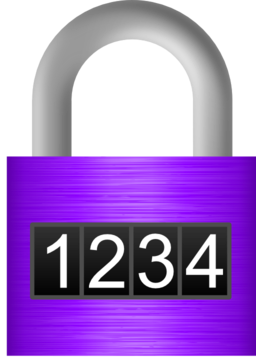# Definition of PermutationA permutation is an arrangement of a set of objects, in which the order of the objects is important.

We often count the number of different possible permutations of a set of objects.

For example, if you want to know the number of different ways you can arrange three students from your class in a straight line, different orders of students will give you different arrangements, so the order is important. If the three students are called Sam, Al and Jasmin, the possible arrangements are:

• Sam, Al, Jasmin
• Sam, Jasmin, Al
• Al, Sam, Jasmin
• Al, Jasmin, Sam
• Jasmin, Sam, Al
• Jasmin, Al, Sam

Permutations of numbers are often used to create the secret codes that are used to open safes and locks. For example, a reordering of the numbers $\{1,2,3,4\}$ might give you the code to open this lock.

An arrangement of a set of objects in which the order doesn't matter is called a combination.

### Description

The aim of this dictionary is to provide definitions to common mathematical terms. Students learn a new math skill every week at school, sometimes just before they start a new skill, if they want to look at what a specific term means, this is where this dictionary will become handy and a go-to guide for a student.

### Audience

Year 1 to Year 12 students

### Learning Objectives

Learn common math terms starting with letter P

Author: Subject Coach
You must be logged in as Student to ask a Question.